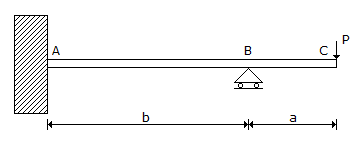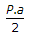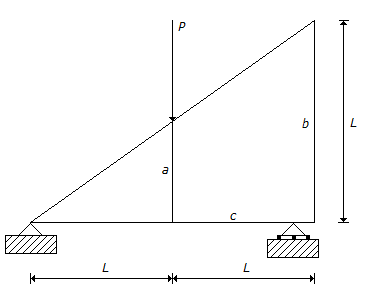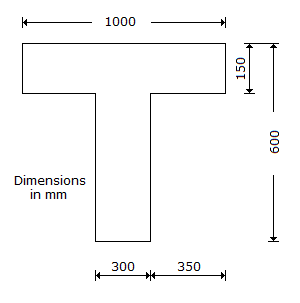# Civil Engineering - GATE Exam Questions

### Exercise :: GATE Exam Questions - Section 6

41.

If the porosity of a soil sample is 20%, the void ratio is

 A. 0.2 B. 0.8 C. 1 D. 0.25

Explanation:

No answer description available for this question. Let us discuss.

42.

The magnitude of the bending moment at the fixed support of the beam is equal to :A. P.a B.C. P.b D. P.(a + b)

Explanation:

No answer description available for this question. Let us discuss.

43.

A soil sample has a void ratio of 0.5 and its porosity will be close to

 A. 50% B. 66% C. 100% D. 33%

Explanation:

No answer description available for this question. Let us discuss.

44.

Which one of the following statements is true with regard to the flexibility method of analysis?A. The method is used to analyse determinate structures B. The method is used only for manual analysis of indeterminate structures C. The method is used for analysis of flexible structures D. The method is used for analysis of indeterminate structures with lesser degree of static indeterminacy.

Explanation:

No answer description available for this question. Let us discuss.

45.

An isolated T beam is used as a walkway. The beam is simply supported with an effective span of 6 m. The effective width of flange, for the cross-section shown in the below figure isA. 900 mm B. 1000 mm C. 1259 mm D. 2200 mm